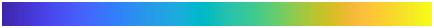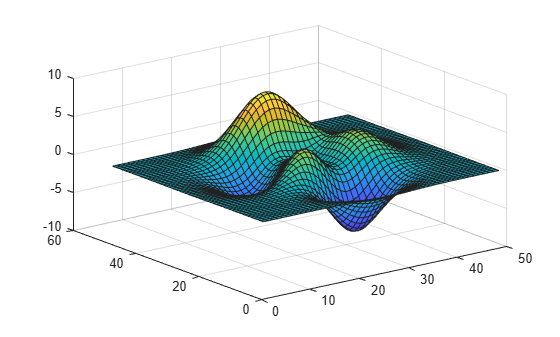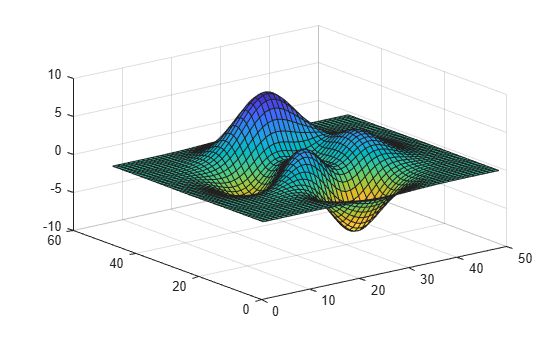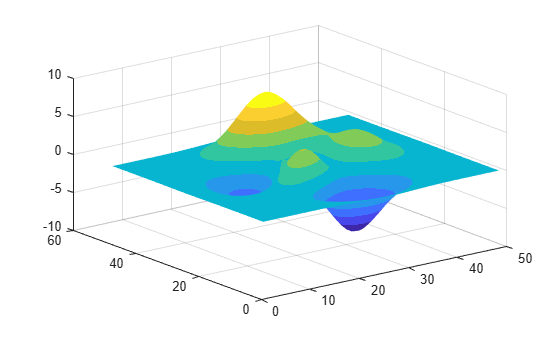# parula

Parula colormap array

•## Syntax

``c = parula``
``c = parula(m)``

## Description

example

````c = parula` returns the parula colormap as a three-column array with the same number of rows as the colormap for the current figure (`gcf`). If no figure exists, then the number of rows is equal to the default length of 256. Each row in the array contains the red, green, and blue intensities for a specific color. The intensities are in the range [0,1], and the color scheme looks like this image.```

example

````c = parula(m)` returns the colormap with `m` colors.```

## Examples

collapse all

Plot a surface with the default parula colormap.

`surf(peaks);`Get the parula colormap array and reverse the order. Then apply the modified colormap to the surface.

```c = parula; c = flipud(c); colormap(c);```Get a downsampled version of the parula colormap containing only ten colors. Then display the contours of the peaks function by applying the colormap and interpolated shading.

```c = parula(10); surf(peaks); colormap(c); shading interp;```## Input Arguments

collapse all

Number of colors, specified as a nonnegative integer. The default value of `m` is the number of colors in the current figure's colormap. If no figure exists, the default value is `256`.

Data Types: `single` | `double`

## Version History

Introduced in R2014b

expand all